# How to calculate days since or until date in Excel

Are you stuck with calculating how many days there are since a certain date or until date? This tutorial will teach you an easy way to add and subtract days from date in Excel. With our formulas you can quickly calculate 90 days from date, 45 days before date, and count whatever number of days you need.

Calculating days from date sounds like an easy task. However, this generic phrase can imply many different things. You may want to find a given number of days after date. Or you may wish to get the number of days from a certain date until today. Or you may be looking to count days from date to date. In this tutorial, you will find solutions to all these and a lot more tasks.

## Days from/before date calculator

Want to find a date that occurs 60 days from a specific date or determine 90 days before date? Supply your date and the number of days in the corresponding cells, and you will get the results in a moment:

## How many days since / until date calculator

With this calculator, you can find how many days are left to a certain date, for example your birthday, or how many days have passed since your birthday:

Tip. To find out how many days there are from date to date, use the Days Between Dates Calculator.

## How to calculate days from date in Excel

To find a date that is N days from a certain date, just add the required number of days to your date:

Date + N days

The key point is to supply the date in the format that Excel understands. I'd suggest using the default date format or convert a text-date to a serial number representing the date with DATEVALUE or explicitly specify year, month and day with the DATE function.

For example, here's how you can add days to April 1, 2018:

90 days from date

`="4/1/2018"+90`

60 days from date

`="1-Apr-2018"+60`

45 days from date

`=DATEVALUE("1-Apr-2018")+45`

30 days from date

`=DATE(2018,4,1)+30`

To get a more universal days from date formula, enter both values (source date and the numbers of days) in separate cells and reference those cells. With the target date in B3 and the number of days in B4, the formula is as simple as adding up two cells:

`=B3+B4`

As plain as it could possibly be, our formula works just perfectly in Excel: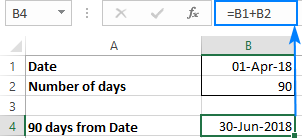With this approach, you can easily calculate the expiry or dues dates for a whole column. As an example, let's find 180 days from date.

Supposing you have a list of subscriptions that expire in 180 days after the purchase date . With the order date in B2, you enter the following formula in, say C2, and then copy the formula to the entire column by double-clicking the fill handle:

`=B2+180`

The relative reference (B2) forces the formula to change based on a relative position of each row: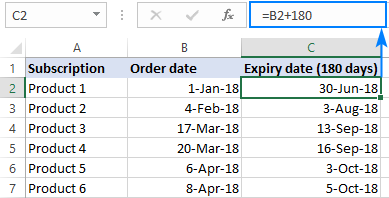You can even calculate a few intermediate dates for each subscription, all with a single formula! For this, insert a couple of new columns and indicate when each of the dates is due (please see the screenshot below):

• 1st reminder: 90 days from purchase date (C2)
• 2nd reminder: 120 days from purchase date (D2)
• Expiration: 180 days from purchase date (E2)

Write the formula for the first cell that calculates the 1st reminder date based on the order date in B3 and the number of days in C2:

`=\$B3+C\$2`

Please notice that we fix the column coordinate of the first reference and the row coordinate of the second refence with the \$ sign so that the formula copies correctly to all other cells. Now, drag the formula rightwards and downwards until the last cells with data, and make sure it calculates the due dates in each column appropriately (please notice that the second reference changes for each column while the first reference is locked to column B):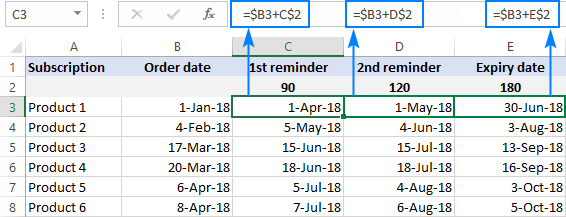Note. If the results of your calculations are displayed as numbers, apply the Date format to the formula cells to have them displayed as dates.

## How to calculate days before date in Excel

To find a date that is N days before a certain date, perform the arithmetic operation of subtraction instead of addition:

Date - N days

As with adding days, it is important that you enter the date in the format understandable to Excel. For example, that's how you can subtract days from a given date, say from April 1, 2018:

90 days before date

`="4/1/2018"-90`

60 days before date

`="1-Apr-2018"-60`

45 days before date

`=DATE(2018,4,1)-45`

Naturally, you can enter both values in individual cells, say date in B1 and the number of days in B2, and subtract the "days" cell from the "date" cell:

`=B1-B2`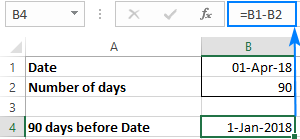## How to count days until date

To calculate the number of days before a certain date, subtract today's date from that date. And to supply the current date that updates automatically, you use the TODAY function:

Date - TODAY()

For example, to find how many days are left until January 31, 2018, use this formula:

`="12/31/2018"-TODAY()`

Or, you can enter the date in some cell (B2) and subtract today's date from that cell:

`=B2-TODAY()`

In a similar manner, you can find difference between two dates, simply by subtracting one date from another.

You can even concatenate the returned number with some text to create a nice-looking countdown in your Excel. For example:

`="Just "& A4-TODAY() &" days left until Christmas!"`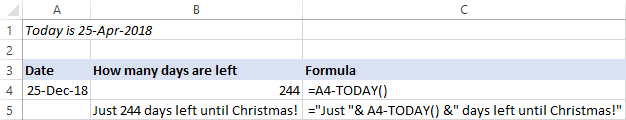Note. If your count days formula shows a date, set the General format to the cell to display the result as a number.

## How to count days since date

To calculate how many days have passed since a certain date, you do the opposite: subtract the date from today:

TODAY() - Date

As an example, let's find the number of days since your last birthday. For this, enter your date in A4, and subtract the current date from it:
`=A4-TODAY()`

Optionally, add some text explaining what that number is:

`=TODAY()-A4 &" days since my birthday"`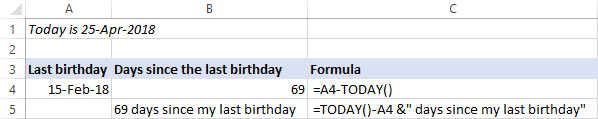## How to calculate working days from date

Microsoft Excel provides 4 different functions to calculate weekdays. The detailed explanation of each function can be found here: How to calculate weekdays in Excel. For now, let's just focus on practical uses.

### Calculate N business days from/before date

To return a date that is a given number of working days ahead of or prior to the start date that you specify, use the WORKDAY function.

Here are a couple of formula examples to get a date that occurs exactly N business days from a certain date:

30 business days from April 1, 2018

`=WORKDAY("1-Apr-2018", 30)`

100 working days from the date in A1:

`=WORKDAY(A1, 100)`

To find a date that occurred a specified number of business days before a given date, supply the days as a negative number (with the minus sign). For example:

120 business days before April 1, 2018

`=WORKDAY("1-Apr-2018", -120)`

90 working days before the date in A1:

`=WORKDAY(A1, -90)`

Or, you can enter both values in predefined cells, say B1 and B2, and your business days calculator can look something similar to this:

Workdays from a given date:

`=WORKDAY(B1, B2)`

Workdays before a given date:

`=WORKDAY(B1, -B2)`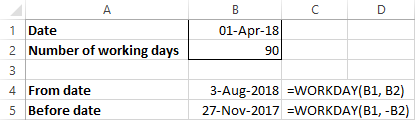Tip. The WORKDAY function calculates days based on the standard working calendar, with Saturday and Sunday as weekend days. If your working calendar is different, then use the WORKDAY.INTL function that allows specifying custom weekend days.

### Count business days since/until date

To return the number of days between two dates excluding Saturdays and Sundays, use the NETWORKDAYS function.

To find out how many working days are left until a certain date, supply the TODAY() function in the first argument (start_date) and your date in the second argument (end_date).

For example, to get the number of days until the date in A4, use this formula:

`=NETWORKDAYS(TODAY(), A4)`

Of course, you are free to concatenate the returned count with your own message like we did in the above examples.

For instance, let's see how many business days are left until the end of 2018. For this, enter 31-Dec-2018 in A4 as a date, not text, and use the following formula to get the number of working days until this date:

`="Only "&NETWORKDAYS(TODAY(), A4)&" work days until the end of the year!"`

Wow, only 179 working days are left! Not as many as I thought :)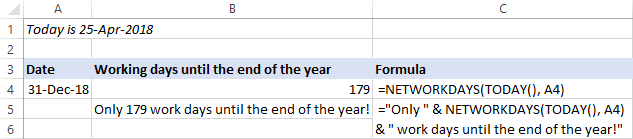To get the number of business days since a given date, reverse the order of arguments - enter your date in the first argument as the start date and TODAY() in the second argument as the end date:

`=NETWORKDAYS(A4, TODAY())`

Optionally, display some explanatory text like this:

`=NETWORKDAYS(A4, TODAY())&" work days since the beginning of the year"`

Only 83 working days… I thought I had worked at least 100 days this year already!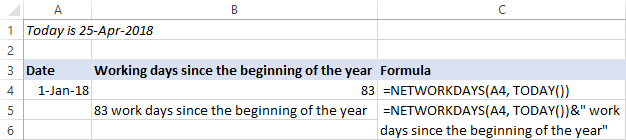Tip. To specify your own weekends other than Saturday and Sunday, use the NETWORKDAYS.INTL function.

## Date and Time Wizard - quick way to calculate days in Excel

This wizard is kind of Swiss army knife for Excel date calculations, it can compute almost anything! You just select the cell where you want to output the result, click the Date & Time Wizard button on the Ablebits Tools tab and specify how many days, weeks, months or years (or any combination of these units) you want to add to or subtract from the source date.

As an example, let's find out what date is 120 days< from date in B2: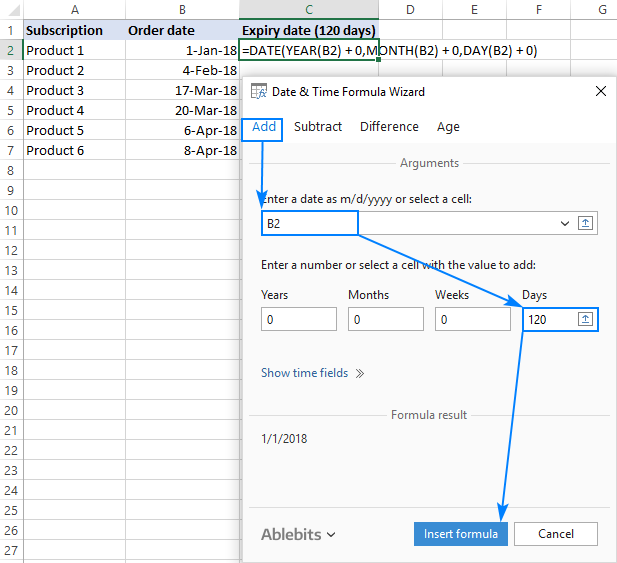Click the Insert formula button to enter the formula in the selected cell, and then copy it to as many cells as you need: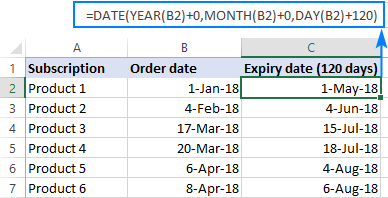As you may have noticed, the formula built by the wizard is different from the ones we've used in the previous examples. It's because the wizard is designed to calculate all possible units, not only days.

To get a date that occurred N days before a certain date, switch to the Subtract tab, input the source date in the corresponding box, and specify how many days you want to subtract from it. Or, enter both values in separate cells, and get a more flexible formula that recalculates with every change you make to the original data: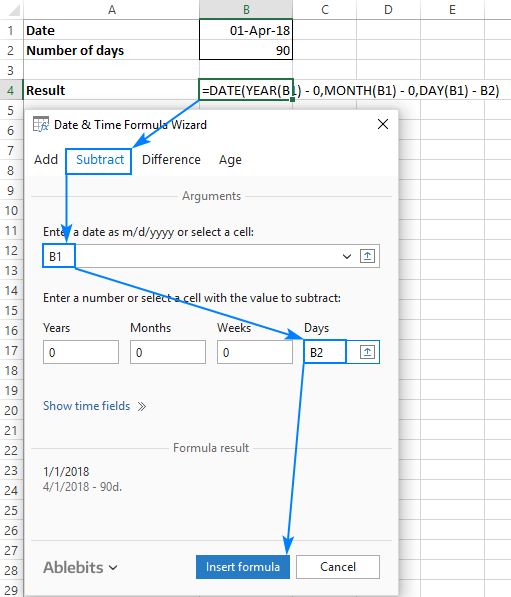## Date Picker - calculate days in drop-down calendar

There exist a great number of third-party drop-down calendars for Excel, both free and paid. All of them can insert a date into a cell with a click. But how many Excel calendars can also calculate dates? Our Date Picker can!

You simply select a date in the calendar and click the Date Calculator icon or press the F4 key: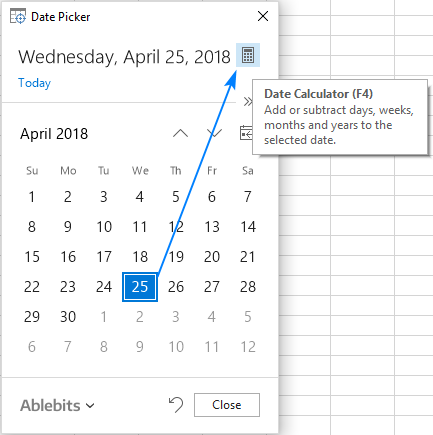Then, click the Day unit on the preview pane and type the number of days to add or subtract (you choose which operation to perform by clicking the plus or minus sign on the input pane).

Finally, press the Enter key to insert the calculated date into the currently selected cell or press F6 to display the date in the calendar. Alternatively, click one of the buttons shown in the image below. In this example, we are calculating a date that is 60 days from April 1, 2018: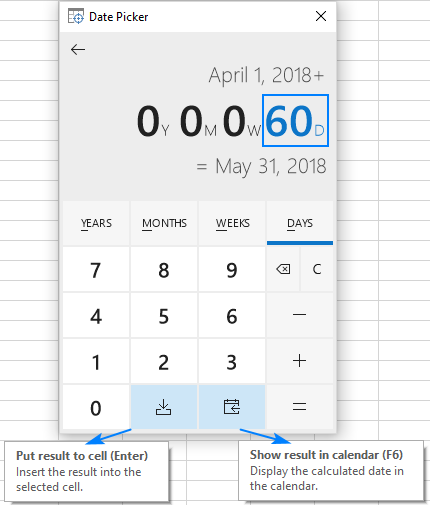That's how you find days from or before a certain date in Excel. I have a closer look the formulas discussed in this tutorial, you are welcome to download our sample workbook to Calculate Days from Date. I thank you for reading and hope to see you on our blog next week!

## You may also be interested in

1. I need to calculate how many days since a date and I have used ,today()- a2 (where the date is recorded in the spreadsheet), however I need this number to stop going up once another cell is marked as complete, is that possible?

• Hi! I’m not sure I got you right since the description you provided is not entirely clear. However, it seems to me that the formula for cell C2 will work for you:

=IF(B2="Complete",MAX(\$C\$1:C2),TODAY()-A2)

2. Dear sir,

I've had an inquiry, so I'm attaching the date below. I need the day of the month. You can see the total number of days from check-in to check-out.

SL NO Name check in Check out DAYS DAYS Jan-23 Feb-23 Mar-23 Apr-23 May-23 Jun-23 Jul-23 Aug-23 Sep-23 Oct-23 Nov-23 Dec-23
1 LABOUR 1 4/10/2023 5/16/2023 1M6d 36
2 LABOUR 2 4/10/2023 5/16/2023 1M6d 36
3 LABOUR 3 3/30/2023 5/16/2023 1M16d 47
4 LABOUR 4 3/30/2023 5/16/2023 1M16d 47

3. Hi
I need once formula for accounting related.

We are releasing the payments in multiple times against the one Invoices - I need difference between invoice date to payment date.

One invoices to multiple payments gap dates

• Hi! I think you will find the answer to your question in the article above.

4. I need to track 30 days +/- 14 days from X date, and then an additional 3 months from that day +/- 30 days. What is the best way to do this?

5. i want to create a formula for days since an injury on a work injury report. i have a list of injuries by date, i want the formula to read the last date and be able to have a days since incident.

• Hello!
You can find the latest date with the MAX function. If you need to find the largest date for a particular person, then use the MAXIFS function. How to calculate the date difference in various ways - read this article on our blog.

• Hi there I have a formula in BK+42 which gives me 42 days after a certain date. But when I drag the formula down to populate in all cells in that column it also adds a random date where no date has been enetered into colum B yet? How to disable this

6. i want to calculate number of days left until zero day. for instance employee is given 20 days leave that number should be minus today(everyday) until zero day when the leave expires. please help me out.

7. Hello,

I am looking for formula that will calculate days from and to a date from an inputted day, August 31st. I would have date on the line and it would need to calculate the days since the fixed date if its past August 31st and calculate from August 31st until that line item if it is in the future. For example: Line 7 has April 31st - the calculation should say 120 days - If line 8 has November 31st it would say 240 days. Is that possible?

• Hi!
Use the DATE function to get the date you need. If I understand your task correctly, try the following formula:

=IF(A1<DATE(YEAR(A1),8,31),A1+120,A1+240)

Hope this is what you need.

8. Hello
I am trying to create a function that I would add the start date in a cell in column B for example. In column D I will have Calendar Days, and in column E I will have workdays.
I would like a formulae to return a date value in column C adding to the start date (in column B) either the number figure in column D or the number figure in column E depending on which cell is populated.
It will be either calendar days or work days number to take into account.
Cheers

• Hello!
If the days are in either column D or column E, then you can simply sum the cells.

=B1+E1+D1

Or use the IF function.

=IF(E1<>"",B1+E1,B1+D1)

9. I have a question, how can I calculate the formula with the following details in one report

I need to have the number of days delayed from the planned end date to actual end date
some of the cell info doesn't have any end date yet since is still in progress.

example: Planned end date Jun 13, 2022 versus Actual End date June 14, 2022
Planned End date June 14, 2022 versus task is still in progress

can this be combine in one formula?

• Hello!
If I understand correctly, if the end date is not specified, then you need to use the current date using the TODAY function.

=IF(B1>A1,B1-A1,TODAY()-A1)

Hope this is what you need.

10. how to calculate (today date -28 day) which date to be .........

• Hi!

11. I have a Start and end date. I got the formula to get the no. of days until today however how do I end this days count when I reach the end date?
Eg. Start dtd 1st April 22 - End dtd 31st July 22.
From 1st Apr until today is 23 days. Every day the days gets updated until the end date.

• Hi!
Use the IF function when the current date is greater than the end date.

=IF(B1 > A1,B1-A1,0)

Hope this is what you need.

• Thank you. Sorry I think I was not very clear with my question. Let me be a bit more elaborate: In the below example Campaign started on 1st Apr and will end on 30 June. Campaign to run for 91 days. As of today 28 campaign days completed which is the last column (I have used the formula to to automatically counts the days). I want to know the formula to use in the last column wherein the day it reaches 91 days the counting should stop and stay at 91 and not get updated to 92 the next day.

Start Date End Date Total Campaign days Completed Campaign Days as of today
1 April 2022 30 June 2022 91 28

• Hi!

=IF(B1 > TODAY(),TODAY()-A1,B1-A1)

• This one did work. Thanks a lot. God Bless

12. I have tried almost every formula and not 1 has worked. I am simply trying to have a date in cell A1 and in cell A2 have it print the total days since that date. So for example if the date in cell A1 was 01/01/2020, and Todays date is 01/02/2020. A2 would print "1"
I keep getting a return of a random date in the year 1900 or ###### with every formula you provided.

• Hello!
If your date format is MM/DD/YYYY then you get the correct result. Please have a look at this article - Default date format in Excel. If this is not what you wanted, please describe the problem in more detail, and what formula you are using.

13. Hi,

Is there a formula on counting the arrear days from the last payment to today's date?
For example I have a lease schedule and the customer missed a payment multiple times.
What will be the formula if the transaction amount is zero, count the days from the last payment to current date? Thanks!

• Hi!
Have you tried the ways described in this blog post? If they don’t work for you, then please describe your task in detail.

14. I am trying to create a running flight tracker where I have my flights tracked for each day. Column A as my date the event occurred, column C is the mode flown. I am trying to establish a box which will compute how many days it has been since the last time a mode was flown. (Ie: if column A is 21-March and column C is NVG, the box would calculate 5. If an entry was made today in column A as 26-March and column C said NVG that box would revert to 0.)

I understand I would need to reference todays date versus the date in column A, I would also need to reference column C against Column A to establish the mode of flight to determine if it is applicable or not. I have 3 modes entered for different days D, N, & NVG. I need the formula to deduce that the applicable date references the correct mode of flight and then determine how many days since current date it has been since that mode was entered.

15. Hi. I'm in the mortgage business. When we issue a mortgage pre-approval, it is only good for 120 days from the time we pull credit.
I'm looking for a formula that will add 120 days to the date I pull credit and display the number of days left before we reach the 120-day expiration date...

Example: 1/1/2022+120 days = 46 days remaining until expiration from today's date of 3/16/2022

• Hi!
All the information you need is in the article above.

=DATE(2022,1,1)+120-TODAY()

Hope this is what you need.

16. HI there,

I'm trying to create a template whereby I need the number of days calculated from a manual date entered which I understand is =today()-a2

What I am trying to achieve is have the value return a "0" value where the formula is when there is no date value entered.

I tried using =if(a2,0,0)today()-a2

This obviously returned an Error, can you please assist me with this formula?

Cheers, Kristen

• Hello!
You can use this formulas with IF function:

=IF(A2<>"",TODAY()-A2,0)

or

=IF(NOT(ISBLANK(A2)),TODAY()-A2,0)

Hope this is what you need.

• HI Alex,

The second solved it!

Thank you SO much!!

Cheers :)

17. can please tell me which formula I should use on 12-Feb-2022.

now I need number 28 days

• Hi!
Sorry, it's not quite clear what you are trying to achieve.

18. I have days and time in a column..I need to count how many are less than or equal to 3 days? How many are greater than 3 days and less than or equal to 5 days?

Thank you

• Hello!
Here is the article that may be helpful to you: COUNTIF formulas for dates.
I don't know how your data is written so I can't give an example formula

19. Kindly help to below table no of days calculation. incase between dates are missing last date minus before date.
sample table below mentioned required all results in one formula.

date 1 date 2 date 3 date 4 date 5 date 6 Ans
01/01/2022 03/01/2022 08/01/2022 17/01/2022 21/01/2022 23/01/2022 2
01/01/2022 03/01/2022 08/01/2022 23/01/2022 15
01/01/2022 23/01/2022 22
01/01/2022 03/01/2022 08/01/2022 Nil
01/01/2022 17/01/2022 21/01/2022 Nil
01/01/2022 03/01/2022 08/01/2022 17/01/2022 21/01/2022 Nil

• Hi!
What do you want to calculate exactly? Your question is not entirely clear, please specify.

20. THANKS, A LOT FOR YOUR LESSON ON THIS MEDIUM.
I NOW USE THIS FORMULA AND IT IS WORKING GOOD
IF(TODAY()-A2<1,"", IF(TODAY()-A2<=28,"POTENTIAL LOSSES", ""))

21. I have this formula problem.
The issue am have is as follow.
Cell A2 contain next appointment date (7/13/2021). If a client missed the appointment day he will become POTENTIAL LOSSES for the next 28 days after the appointment day and on the 29th day he become LTFU( Lost to follow up).
I used this formula =IF(TODAY()-A2<=28,"POTENTIAL LOSSES","")
it is returning even future date such as 8/30/2021 as "POTENTIAL LOSSES" where as it should give it as Blank cell and it is not even return "POTENTIAL LOSSES" a day after missing the appointment date whereas it should return "Potential losses" till 28th day and change to lost to follow up on the 29th day.

• Hello!
Formula = IF(TODAY()-A2<=28, "POTENTIAL LOSSES", "") returns "POTENTIAL LOSSES" for me. It works correctly.

• Thank you for your reply. The problem is the future date from today is returning as POTENTIAL LOSSES which should return BLANK-like 29 days after the appointment day. I don't know what is the cause, sir. I will appreciate it if you run it and put future say
13/8/2021 in A2 and see what it will return.

• How do you use the future date in the formula? Where do you insert it? The TODAY function returns today's date. If you use a cell with the date 8/30/2021 instead of TODAY, the formula returns "".

22. Want to calculate no. Of days with TRUE or FALSE if days is more then 7 day's after week off or holiday...what formula need to write

23. I want to work out a date in the past from a certain date and certain number of days before or after. So I want the date 15 days before a specific date, but I do not want to add the Sunday, the public holiday and the school holiday. So I have the calendar for the year, a day is assigned a zero or a 1. All days on which the school will be open as well as a Saturday will be a 1. Any other day on which there will not be school like a school holiday, Sunday or public holiday will be a zero. I am missing something here. PLease assist, thanks

• Hello!
You can calculate the number of days between two dates, taking into account the weekend. You can also use your own list of weekends and holidays.

24. Hello,

I need to calculate days till something is due and then continuing counting how many days overdue (e.g. -3 days late). I have a formatted the due date and then in the column overdue what formula do i put?

25. 30 days late on payment and I want to make it update each date they are late.

Ex: 30days late making the next day(s) go up each day automatically So I don't have to do it myself each day

• Hello Harry!
Please describe your problem in more detail. Write an example of the source data and the result you want to get. It’ll help me understand it better and find a solution for you. Thank you.

26. Hi: is there a modification to use to exclude holidays when calculating workdays using this formula:
=workday(A1, -2)

27. Bonjour,

J'aimerais trouver une formule pour calculer le nombre de jours depuis une date jusqu'aujourd'hui, SVP.
Je n'ai que la date entrée donc les formules de DATEDIF et DATEVAL ne peuvent pas marcher.
Ex: j'ai un dossier de 2017 qui n'est pas fini, que dois-je faire pour avoir le nombre de jours à partir de 2017 jusqu'aujourd'hui?

Thanks a lot

29. HI,
Would like to calculate the due days based on the present days.

30. What is the date four days before November first 2018 without counting the weekend

• Hi Brenda,

The below formula says it's October 26, 2018:

=WORKDAY("1-Nov-2018", -4)

Please remember to apply the Date format to the formula cell for the result to display correctly.

• I NEED A EXCEL FORMULA TO calculate 15 days free storage

31. I have a monthly report but need to count only daily data. I don't know to use Today option if formula is =COUNTIF(A1:A50,"Vacation")=TODAY(). Please help

• IvTe:
If you can use COUNTIFS in your version of Excel then I believe this formula is what you're looking for.
=COUNTIFS(A1:A50,"=VACA*",B1:B50,"<="&TODAY())
Where the word "VACATION" is in A1:A50 and the Date is in B1:B50. Note this formula uses a wildcard so "Vaca" is counted, too.